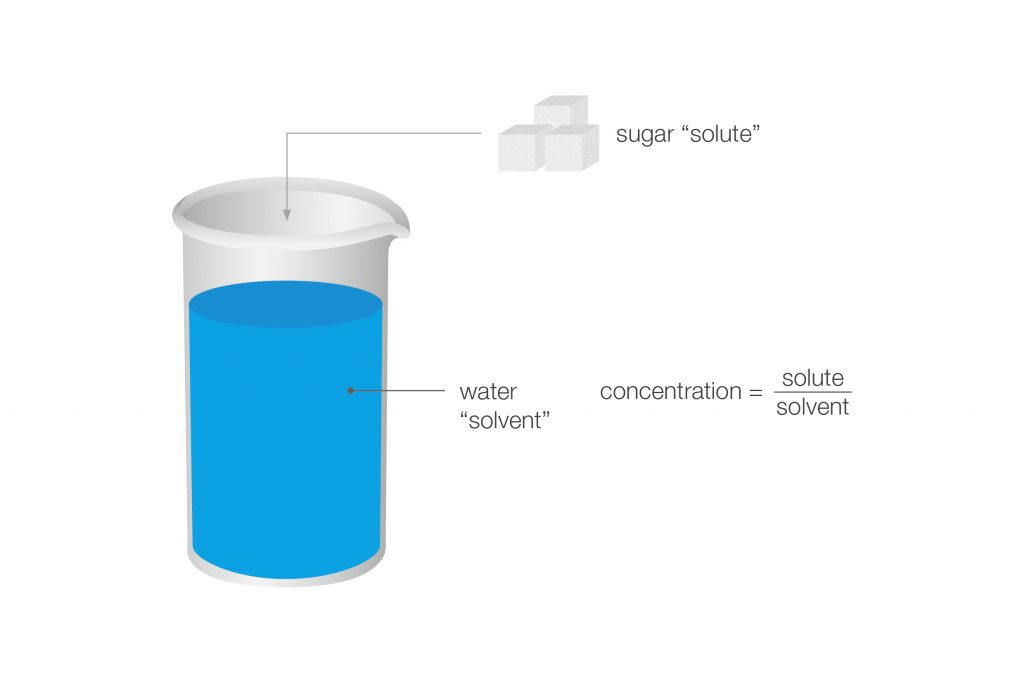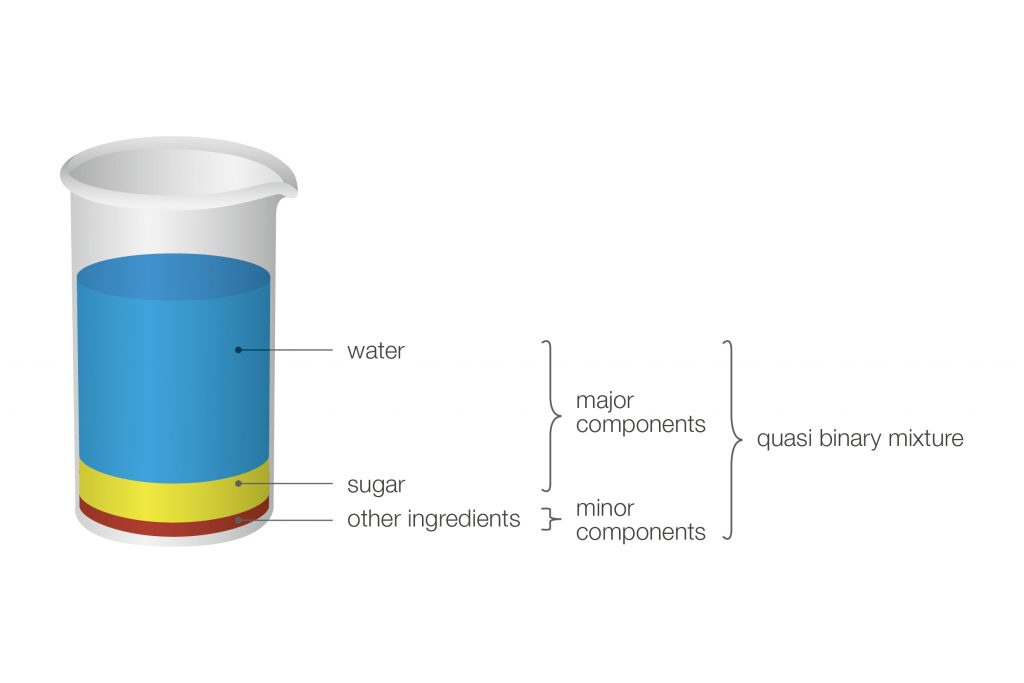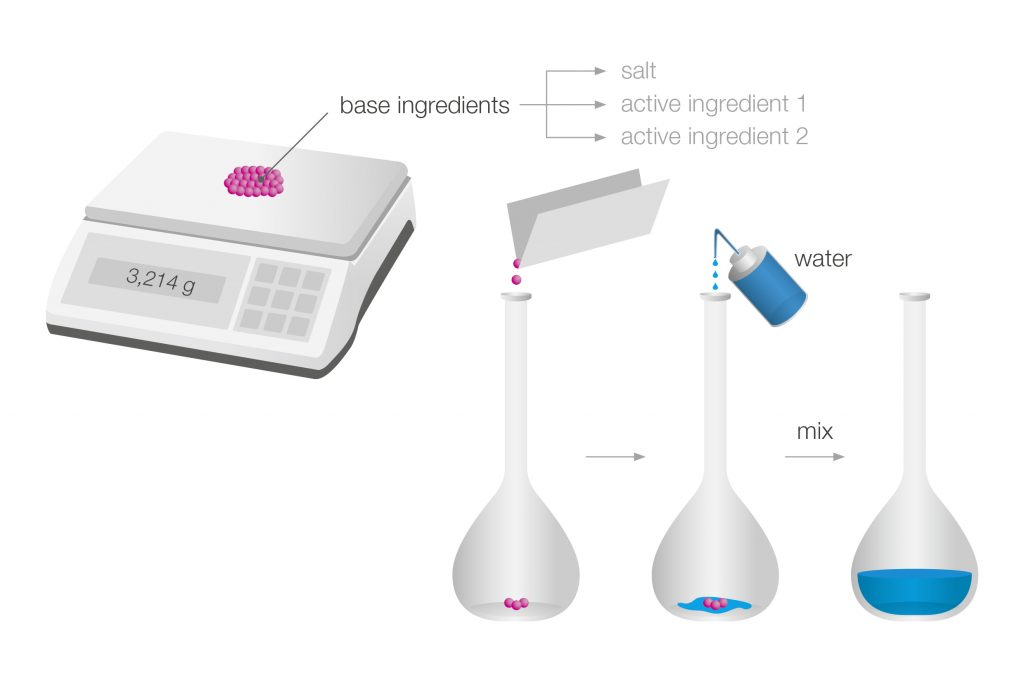28 Rates

# Concentration determination by means of density measurement

If there is a relation between the concentration of a substance in a solution and the density of the solution, the concentration can be determined by measuring the density of the solution. This article focuses on binary solutions, the different possibilities to measure their concentration, and highlights the most important units for the description of these mixtures.

## General aspects

The density of liquids changes if ingredients change. For example: a soft drink containing sugar will have a higher density than a diet soft drink. So, sugar not only increases a liquid’s density, it can also be inversely measured by knowing the liquid’s density in a given liquid type. Using this correlation provides useful information in the production of many beverages.Figure 1: By adding a substance to a solvent, the density of the mixture will be different from the density of the pure solvent. Density can therefore be used to determine the concentration of a substance (“solute”) in a solution (e.g. water).

Figure 2: Mixing two samples with known densities A and B results in a mixture with a density that lies between value A and B.

### Binary and quasi-binary mixtures

Two-component mixtures are called binary mixtures. The density of the mixture is a function of its composition. Thus, the density value of a binary mixture can be used to calculate its composition with the aid of concentration tables.

Typical two-component mixtures are e.g. alcohol-water solutions, sugar-water solutions, salt-water solutions, and acids or bases dissolved in water.

Concentration determination is also possible with so-called quasi-binary mixtures. These are mixtures containing two major components and some additional ingredients which are present in very small concentrations compared to the two main components. Due to their small impact on the bulk density these additional ingredients can be ignored.

Concentration determination is also possible if a mixture contains several components and only one of them varies while all other ingredients are constant.Figure 3: The major components of regular soft drinks are water and sugar (saccharose), all other ingredients, such as flavors or food coloring, are present in an insignificant amount. Therefore, soft drinks are considered sugar-water solutions and density measurement is used to determine the sugar content.Figure 4: For the production of infusions several base ingredients including salts and active ingredients are precisely weighed according to a recipe. In a second step this mixture is diluted with water. The concentration can be controlled by means of density measurement.

## Density measurement using a digital density meter

Countless analytical instruments prevail in today’s laboratories for quality and production control. However, not all of them are as easy, fast, and significant as a digital density meter.

Modern density meters require very little sample, do not change the sample’s composition, are not operator-dependent, reduce chemical consumption, and perform at the utmost precision.

The sample is introduced into a U-shaped tube that is electronically excited to oscillate at its characteristic frequency. The characteristic frequency changes depending on the density of the filled sample. Via a precise measurement of the characteristic frequency the density of the sample is determined. Due to the high temperature dependency of density, the measuring cell has to be accurately temperature-controlled.

Modern high-precision density meters additionally provide a viscosity correction and a reference oscillator to enable accurate results over a large range of densities, temperatures, and viscosities. The U-tube method is used to measure the true density ρ of fluids.

Figure 5: Oscillating U-tube principle (U-tube filled with air or water)

### Conversion of density into concentration units

The density of a mixture can be converted into a concentration unit using a suitable concentration table or, more generally, a conversion formula. Nearly all binary solutions can be characterized by using density-related functions and concentration tables. These tables may either come from literature or individual experimental data.

The concentration of the solutions or mixtures is often expressed in terms of the solution’s percentage composition on weight or volume basis.

 Quantity Unit Ethanol tables AOAC 60 °F, HM C&E, IUPAC, KAEMPF, OIML, OIML-ITS-90, Proof 60 °F, Canadian Excise Alcohol Table, OIML-Shusei-Do %v/v (Vol.-%), %w/w (%m/m, Gew.-%), °Proof Extract/sugar tables Baumé, Concentration Sugar, Mass Concentration Sugar °Baumé, °Brix, °Plato, g/L, kg/m³ Acid/base tables HCl, HNO3, H3PO4, NaOH, H2SO4 %w/w (%m/m, Gew.-%), mol/L, N API functions API Gravity 15 °C / 20 °C / 29.5 °C / 60 °F, API Density 15 °C / 20 °C / 29.5 °C / 60 °F, API Input Quantity, API Product Group, API Specific Gravity 15 °C / 20 °C / 29.5 °C / 60 °F g/cm³, kg/m³, lb/gal, g/mL

Table 1: Preferred units for different quantities in several industries

In the chemical field concentrations are often reported in %weight/weight or %mass/mass. This is abbreviated as %w/w or %m/m. Generally this means: gram of the component per 100 gram of the solution.

One example: 5 % sulfuric acid means that there is 5 gram of sulfuric acid in 100 gram of the solution. Temperature can be ignored here, since temperature does not have any influence on weight or mass. While most industries use %weight/weight, the alcohol industry mainly reports their concentration in %volume/volume. If you look at a bottle of beer, you will typically see the alcohol content reported in %V/V. This, of course, is a temperature-dependent unit. In the pharmaceutical industry, for example, a disinfection solution may contain 70 % ethanol (V/V). This means that there are 700 mL of ethanol in 1 L solution.

A common concentration unit in the brewing industry for describing the concentration of beer and wort is °Plato or °Balling. This refers to the extract content in the product, typically beer wort, and is more or less the same.

°Proof is another unit used basically in the spirits industry in the United States. It is %V/V of alcohol multiplied by 2 at 60 °F:

$$°Proof ^{60°F} = 2 * P^{60°F}$$

The API, which stands for American Petroleum Institute, came up with their own gravity term ° API Gravity to easily characterize heavy or light petroleum products compared to water: If the API gravity (at 60 °F) of a petroleum product is greater than 10, it is “light” and floats on water, if it is less than 10, it is “heavier” than water and sinks. API gravity is reported in degrees and calculated based on a formula, which includes specific gravity. Depending on the country or norm 60 °F or 15 °C are used as the related temperature. This scale is generally used for most transactions in the United States as well as in refinery practice around the world.

$$API Gravity = {141.5 \over SG} - 131.5$$

Equation 1: Formula to calculate the API gravity of a petroleum product based on the specific gravity SG of a sample

In the chemical industry °Baumé can be used as a unit. It is similar to the API number, using specific gravity SG in a formula.

$$°Bé = {140 \over SG } - 130 \hspace{10mm} SG\leq1$$ $$°Bé = 145 - {145 \over SG } \hspace{10mm} SG>1$$

Equation 2: There are two Baumé formulas, one for liquids that are heavier than water (specific gravity SG > 1) and one for liquids that are lighter than water (specific gravity SG ≤ 1)

In the soft drink industry °Brix is used for degree saccharose or degree extract in the product. °Brix and °Plato are basically identical.

## A non-linear relation: Concentration determination of sulfuric acid

Sometimes a concentration table for a specific substance does not cover the whole concentration range from 0 %w/w to 100 %w/w. One reason might be that the solute is already present in an amount at which no more can be dissolved (“saturation”).

Another reason might be that the density of the solution does not show a linear relation to the concentration value. This is the case with sulfuric acid: When determining sulfuric acid in water, density measurement gives precise concentration readings in a concentration range from 0 % to 87 %. But if the concentration of sulfuric acid is higher (87 % to 100 %), the density increases no more with increasing amounts of dissolved acid.

The density of a sulfuric acid solution does not show a linear relation to the concentration value. The density value shows a maximum at a sulfuric acid concentration of 87 %, resulting in a value that can no longer be correlated to one concentration only. To cover the whole concentration range a second measuring parameter has to be used, e.g. sound velocity (Figure 5).

## Accuracy of the measurement result

The accuracy of the measuring result depends not only on the density accuracy of the measuring instrument but also on the quality of the reference data (i.e. density to concentration table) and the calculation behind the conversion function (e.g. a table or a polynomial function). The more accurate your density measurement is, the more accurate your concentration determination will be.

Example: How to calculate accuracy
A sodium chloride (NaCl) solution is measured with an instrument with an accuracy of 0.000005 g/cm³. Two concentrations of the solution (0.5 %w/w and 1.5 %w/w NaCl) are considered as they span the expected sample concentration range. The densities of both solutions are measured and the difference ∆ between the densities is calculated.

 NaCl 0.5 %w/w ...... 1.0018 g/cm³ 1.5 %w/w ...... 1.0089 g/cm³ Δ ...... 0.0071 g/cm³

The difference ∆ of 1.0 %w/w corresponds to a density change of 0.0071 g/cm³, thus the accuracy of the NaCl solution is: (1.0 x 0.000005)/0.00710 = 0.007 %w/w. This is the average accuracy for the overall range as defined above, and only true assuming the relation of density to concentration is linear.

You can achieve more accurate results with your user table when there are many obtained data pairs (density value and corresponding concentration) with high accuracy. Due to the non-linear behavior of real solutions the accuracy is only valid in a certain concentration range.

### Alternative methods for determining the concentration

Titration: A common disadvantage of titration is that it requires a skilled operator with chemical know-how. A limited concentration range is covered, so the sample has to be diluted accordingly. Further, a special setup depending on each individual sample is needed. Another drawback of titration is the use of aggressive chemicals and the need of costly consumables. These points make this method very time-consuming and not user-friendly.

Pycnometer: A pycnometer is an affordable measuring device that gives highly accurate measurement results. However, to achieve highly accurate results a very skilled operator is needed as well as a very precise balance. Another drawback is the temperature control of the sample of which a large amount (up to 100 mL) is needed. All in all this method is quite time-consuming and it is only possible to determine the apparent density of the sample, so the buoyancy of air has to be compensated.

Hydrometer: Hydrometers are affordable and provide a very fast concentration measurement. However, the scale can easily be misread and a temperature correction is necessary. As is the case with pycnometers, a large sample amount is needed and the hydrometer can break easily.

Hydrostatic balance: Hydrostatic balances are used in special facilities, for example the National Bureau of Standards, because they are incredibly precise and reliable. However, a hydrostatic balance is a huge, complex apparatus with a concrete foundation that needs to be insulated and extensively temperature-controlled. This “instrument” is very expensive and not suitable for industry. Measurements are immensely time-consuming and might take one to two days.

## Conclusion

Determining the concentration of binary mixtures is required whenever a liquid product is produced, traded, or consumed. The basics of binary mixtures are presented in this article, as well as measurement methods and the different units to compare and characterize the products depending on the industry.